### Risk

An event risk of a population over a specified time period can be defined as the number of new events in the follow-up time period divided by the event-free population size at the beginning of the time period,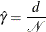where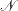is the population size.

For a general population, the subsets (strata) might not be homogeneous enough to have a similar risk. Thus, the risk for each stratum should be computed separately to reflect this discrepancy. For a population that consists of K homogeneous strata (such as different age groups), the stratum-specific risk for the jth stratum in a population is computed as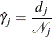where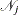is the population size in the jth stratum of the population.

Assuming the number of events,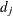, has a binomial distribution, then a variance estimate of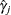is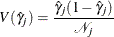By using the method of statistical differentials (Elandt-Johnson and Johnson, 1980, pp. 70–71), the variance of the logarithm of risk can be estimated by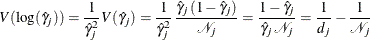#### Normal Distribution Confidence Interval for Risk

A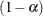confidence interval forbased on a normal distribution is given by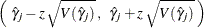where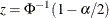is the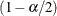quantile of the standard normal distribution.

#### Lognormal Distribution Confidence Interval for Risk

Aconfidence interval for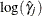based on a normal distribution is given by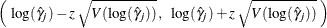whereis thequantile of the standard normal distribution and the variance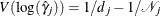.

Thus, aconfidence interval forbased on a lognormal distribution is given by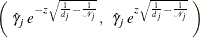#### Confidence Interval for Risk Difference Statistic

For rate estimates from two independent samples,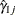and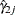, aconfidence interval for the risk difference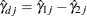is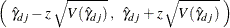whereis thequantile of the standard normal distribution and the variance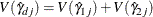#### Confidence Interval for Risk Ratio Statistic

For rate estimates from two independent samples,and, aconfidence interval for the log risk ratio statistic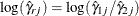iswhereis thequantile of the standard normal distribution and the variance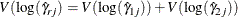Thus, aconfidence interval for the risk ratio statistic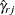is given by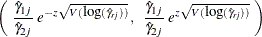#### Confidence Interval for Risk SMR

At stratum j, a stratum-specific standardized morbidity/mortality ratio is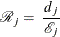where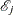is the expected number of events.

With the riskSMR can be expressed as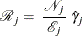Thus, aconfidence interval for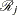is given by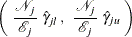where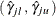is aconfidence interval for the risk.